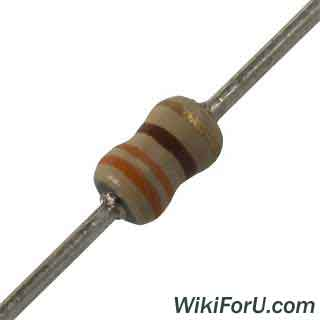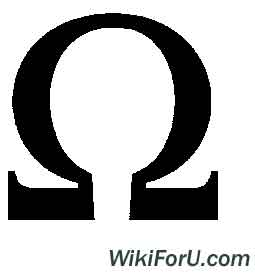## What is Resistance and Resistors | Online Electrical Tutorials

Tuesday, November 22, 2011Resistance - An Electrical Resistor

Resistance:
Resistance of a material is the property which opposes flow of electrons in that conductor. Thus its the opposition of the conductor to the current flowing through the conductor.
Which component has this property is called Resistor. There are some types of Resistors.
Related Articles: Must Have Tools For An Electrician
Unit:The Resistance of a Resistor is measured in Ohms. The symbol of ohms is shown below:Resistance can be measured by Ohm's law or by Wheat Stone Bridge.
According to ohm's law:
V = I.R
R = V/I
Resistance = Voltage/Current
Related Articles: Basic Measurement System And Working

Types of Resistors:
• Fixed Resistors
• Variable Resistors
Fixed Resistors: These are those resistors whose resistance can't be varied. Mean they have fixed resistance. These are sub divided into following types:
1. Carbon Composition Resistors
2. Wire Wound Resistance
3. Carbon Film Resisters
Related Articles: What is Commutation?
Variable Resisters:
These are the resisters whose resistance can be varied. Rheostats and Regulators are examples of Variable Resistors. Variable resisters are widely used components. We use it to start a d.c. shunt motor and in many other electrical applications.
This is a simple overview of Resistance. If you want to know anything advanced, then please ask by commenting.